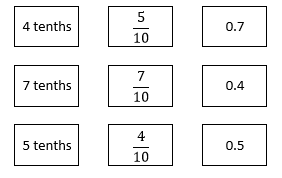# Decimal Fractions

## Objective

Represent decimals to tenths less than one with area models. Convert between fraction, decimal, and unit form.

## Materials and Resources

• Base ten blocks  — 10 one cubes, 10 ten rods, 10 hundred flats, 1 thousand cube

## Common Core Standards

### Core Standards

?

• 4.NF.C.6 — Use decimal notation for fractions with denominators 10 or 100. For example, rewrite 0.62 as 62/100; describe a length as 0.62 meters; locate 0.62 on a number line diagram.

?

• 3.NF.A.2

## Criteria for Success

?

1. Look for and make use of structure to understand the importance of the unit tenths as an extension of the place value system to represent values smaller than one (MP.7).
2. Understand a decimal fraction to be a fraction whose denominator is 10 (or, as explored in later lessons, another power of ten like 100).
3. Understand a decimal number to be a number where each place represents some power of ten (such as tenths, as explored in this lesson).
4. Understand that a decimal point helps to delineate between whole numbers and decimal numbers when writing a number in decimal form.
5. Represent decimals to tenths less than one written in decimal, fraction, or unit form using an area model (MP.5).
6. Convert between fraction form, decimal form, and unit form for some number of tenths less than one.

## Tips for Teachers

?

While “mathematicians and scientists often read 0.5 aloud as ‘zero point five’ or ‘point five,’” refrain from using this language until you are sure students have a strong sense of place value with decimals (NF Progression, p. 15).

#### Fishtank Plus

• Problem Set
• Student Handout Editor
• Vocabulary Package

?

### Problem 1

1. Complete the following sentences. Use base ten blocks to help you.
1. 1 thousand is the same as _______ hundreds.
2. 1 hundred is the same as _______ tens.
3. 1 ten is the same as _______ ones.
2. Use what you notice in (a) to write a similar sentence that starts “1 one is the same as…”

3. Use what you just learned in (a) and (b) above to fill in the missing place value.### Problem 2

a. Imagine the following model as a zoomed-in ones cube. Partition and shade it to represent the following fractions.i.   2 tenths

ii.   3 tenths

iii.   5 tenths

b. Fill out the following table.

 Unit Form Fraction Form Decimal Form 2 tenths 3 tenths 5 tenths

## Discussion of Problem Set

?

• What relationship does 1 tenth have to 1?
• How did your work with decimal fractions like ${{3\over10}}$${{7\over10}}$, or ${{9\over10}}$ prepare you for this lesson?
• Today, we studied decimal numbers and we wrote them in fraction form and decimal form. How are the two forms alike? How are they different?
• What purpose does a decimal point serve?

?

### Problem 1

Match each amount expressed in unit form to its fraction form and decimal form.#### References

EngageNY Mathematics Grade 4 Mathematics > Module 6 > Topic A > Lesson 1Exit Ticket, Question #2

Grade 4 Mathematics > Module 6 > Topic A > Lesson 1 of the New York State Common Core Mathematics Curriculum from EngageNY and Great Minds. © 2015 Great Minds. Licensed by EngageNY of the New York State Education Department under the CC BY-NC-SA 3.0 US license. Accessed Dec. 2, 2016, 5:15 p.m..

Modified by The Match Foundation, Inc.

### Problem 2

Draw an area model to match the decimal.

0.9

0.2

?